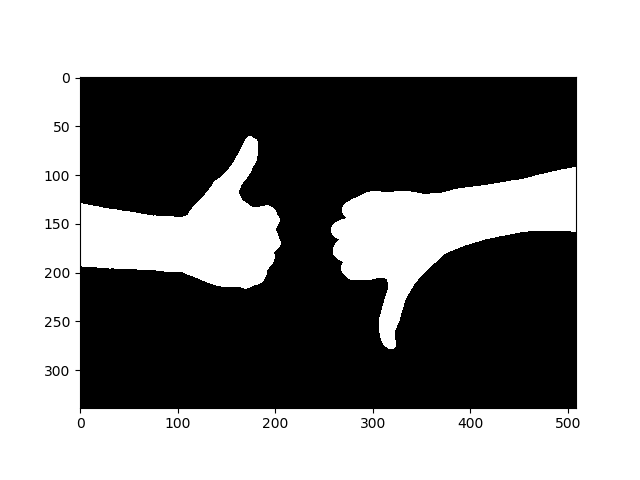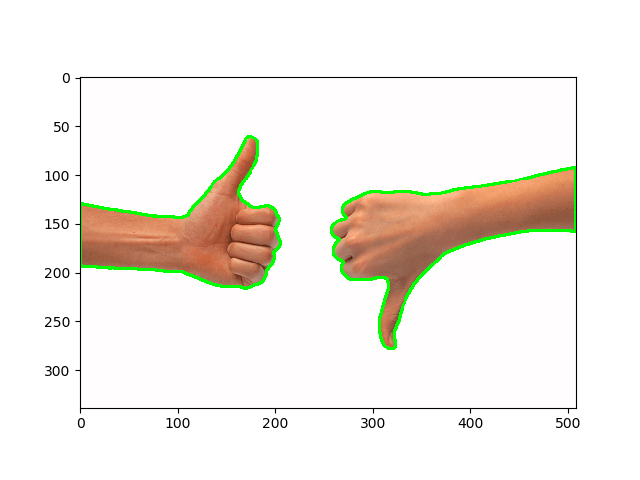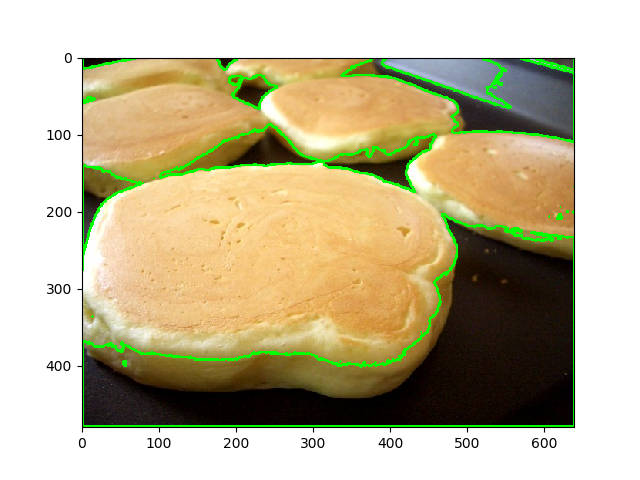# How to Detect Contours in Images using OpenCV in Python

Learning how to detect contours in images for image segmentation, shape analysis and object detection and recognition using OpenCV in Python.
5 min read · Updated may 2023 · Machine Learning · Computer Vision

Disclosure: This post may contain affiliate links, meaning when you click the links and make a purchase, we receive a commission.

A contour is a closed curve joining all the continuous points having some color or intensity, they represent the shapes of objects found in an image. Contour detection is a useful technique for shape analysis and object detection and recognition.

In a previous tutorial, we have discussed edge detection using the Canny algorithm and we've seen how to implement it in OpenCV, you may ask, what's the difference between edge detection and contour detection?

Well, when we perform edge detection, we find the points where the intensity of colors changes significantly, and then we simply turn those pixels on. However, contours are abstract collections of points and segments corresponding to the shapes of the objects in the image. As a result, we can manipulate contours in our programs such as counting the number of contours, using them to categorize the shapes of objects, cropping objects from an image (image segmentation), and much more.

Contour detection is not the only algorithm for image segmentation though, there are a lot of others, such as the current state-of-the-art semantic segmentation using transformers, hough transform, and K-Means segmentation.

For better accuracy, here is the whole pipeline that we gonna follow to successfully detect contours in an image:

• Convert the image to a binary image, it is a common practice for the input image to be a binary image (which should be a result of a thresholded image or edge detection).
• Finding the contours using findContours() OpenCV function.
• Draw these contours and show the image.

Alright, let's get started. First, let's install the dependencies for this tutorial:

``pip3 install matplotlib opencv-python ``

Importing the necessary modules:

``````import cv2
import matplotlib.pyplot as plt``````

We gonna use this image for this tutorial:``````# read the image

Converting it to RGB and then grayscale:

``````# convert to RGB
image = cv2.cvtColor(image, cv2.COLOR_BGR2RGB)
# convert to grayscale
gray = cv2.cvtColor(image, cv2.COLOR_RGB2GRAY)``````

As mentioned earlier in this tutorial, we gonna need to create a binary image, which means each pixel of the image is either black or white. This is a necessity in OpenCV, finding contours is like finding a white object from a black background, objects to be found should be white and the background should be black.

``````# create a binary thresholded image
_, binary = cv2.threshold(gray, 225, 255, cv2.THRESH_BINARY_INV)
# show it
plt.imshow(binary, cmap="gray")
plt.show()``````

The above code creates the binary image by disabling (setting to 0) pixels that have a value of less than 225 and turning on (setting to 255) the pixels that has a value of more than 225, here is the output image:Now, this is easy for OpenCV to detect contours:

``````# find the contours from the thresholded image
contours, hierarchy = cv2.findContours(binary, cv2.RETR_TREE, cv2.CHAIN_APPROX_SIMPLE)
# draw all contours
image = cv2.drawContours(image, contours, -1, (0, 255, 0), 2)``````

The above code finds contours within the binary image and draws them with a thick green line to the image, let's show it:

``````# show the image with the drawn contours
plt.imshow(image)
plt.show()``````

Output image:To achieve good results on different and real-world images, you need to tune your threshold value or perform edge detection. For instance, for a pancakes image, I've decreased the threshold to 127, here is the result:Alright, this is it for this tutorial, if you want to test this on your live camera, head to this link.

Finally, I've collected some useful resources and courses for you for further learning, I highly recommend the following courses:

## Mastering YOLO: Build an Automatic Number Plate Recognition System

Building a real-time automatic number plate recognition system using YOLO and OpenCV library in Python

Happy Coding ♥

Sharing is caring!

### Comment panel